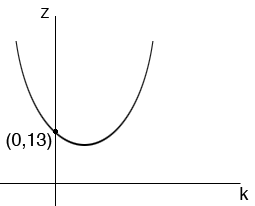SEARCH HOMEMath Central Quandaries & QueriesQuestion from Claire, a parent: Show that the roots of (x - k)(1-3x) + 1 = 0 are real and distinct for all real values of k. Hence, or otherwise, find the range of 9sin^2 r - 6sin r + 13Hi Claire,

I expanded (x - k)(1-3x) + 1 = 0 to obtain a quadratic in x. I then used the general quadratic to solve for x and examined the discriminant, b2 - 4ab. The discriminant is a quadratic in k. The quadratic equation (x - k)(1-3x) + 1 = 0 has distinct real roots of this discriminant is positive.

Try this and see if you can show that the discriminant is positive. If you have trouble write back and tell us what you got for the discriminant and we will try to help.

Harley Weston

Claire wrote back.

Hi Harley

Here are the steps that I managed before getting stuck.

(x-k)(1-3k)+1=0
(x-3x^2-k+3kx+1)=0
3x^2-3kx-x+k-1=0
3x^2-x(3k+1)+(k-1)=0

If the roots are real and distinct then b2-4ac > 0

(3k+1)2 - 4(3)(k-1)
= 9k2-6k+13

Thank you.

Regards
Claire

Claire,

That's exactly what I got but you should say

If b2-4ac > 0 then the roots are real and distinct.

I agree 9k2-6k+13 cant be factored so I solved 9k2-6k+13 = 0 using the general quadratic again. This time the discriminant is negative so the roots are not real!

Consider the function

z = 9k2-6k+13

Its graph in the kz-plane never crosses the k-axis since 9k2-6k+13 = 0 has no real solution. When k = 0 the value of z is 13 which is positive. Hence 9k2-6k+13 is positive for some value of k and to become negative it would have to cross the k-axis, which it doesn't. Hence 9k2-6k+13 is positive for all values of k.

The graph of z = 9k2-6k+13 looks something likeHarleyMath Central is supported by the University of Regina and The Pacific Institute for the Mathematical Sciences.